## Example Questions

### Example Question #1 : How To Find The Perimeter Of A Right Triangle

Max left his house and drove 10 miles north to work.  From work, he then drove 24 miles east to pick up his daughter from the airport. What is the shortest distance Max can to drive to get back home?

20

34

12

26

26

Explanation:

It is easiest to solve this using special triangle formulas. From the description, we know this is a right triangle with legs of 10 and 24. 10 and 24 are multiples of the special triangle with side ratio 5 :12 :13.

Since we multiplied 5 and 12 by 2 to get 10 and 24, respectively, we compute the diagonal to be 2 * 13 = 26.

Using the Pythagorean Theorem, a2 + b2 = c2, is also an acceptable approach to solving this problem.

### Example Question #1 : How To Find The Perimeter Of A Right Triangle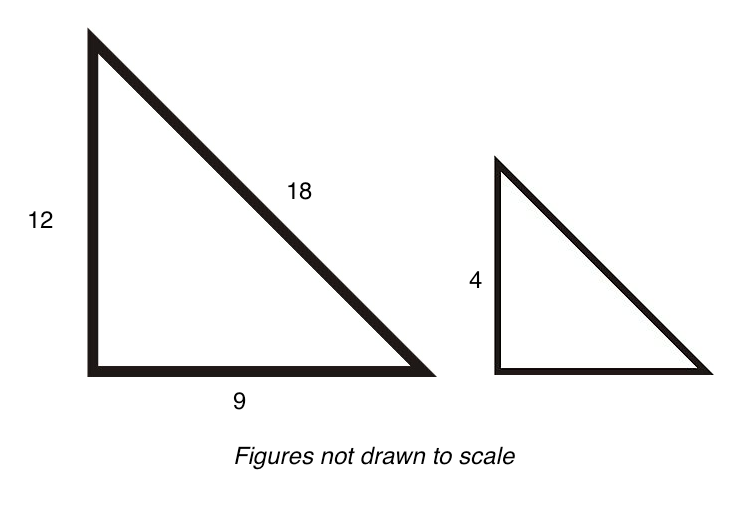These two triangles (not drawn to scale) are similar triangles.

What is the perimeter of the triangle above?

39

19

10

9

13

13

Explanation:

These two triangles are similar triangles.  The triangle on the right is one-third the size of the triangle on the left.  We know this by comparing the bottom legs of each triangle.

3/9 = 1/3

Given this, all the other legs of the triangle on the right will be 1/3 the size of their corresponding legs on the left.

12 * 1/3 = 4
18 * 1/3 = 6

Now we know the lengths of the three sides, we simply add them together to receive our answer.

4 + 6 + 3 = 13

An alternate way to do this problem would be to take 1/3 the perimeter of the triangle on the left.

12 + 18 + 9 = 39

1/3 * (39) = 13

Either method will enable you to arrive at the same solution.

### Example Question #66 : Triangles

Triangle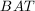is defined by the coordinates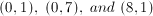. What is the perimeter of triangle?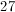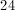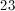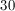Explanation:

These three points form a right triangle on the Cartesian coordinate system. The perimeter is comprised of the length from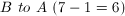; the length from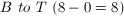; and the length of the hypotenuse between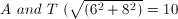.

The perimeter is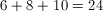.

### Example Question #67 : Triangles

Quantity A: The perimeter of a right triangle with two short sides of 10 and 24.

Quantity B: The area of a right triangle with two short sides of 10 and 12.

Quantity B is greater.

Quantity A is greater.

The relationship cannot be determined from the information given.

The two quantities are equal.

The two quantities are equal.

Explanation:

In order to find the perimeter of the right triangle in Quantity A, we must find the length of the hypotenuse. We know that by the Pythagorean theorem, the hypotenuse is equal to the square root of (102 + 242) = 100 + 576 = 676. The square root of 676 is 26, so the perimeter of the right triangle is 10 + 24 + 26 = 60.

We could also have noticed that the right triangle is similar to a 5-12-13 right triangle with a dilution ratio of 2, since the 10-24 sides are twice the length of the 5-12 sides.

The area of the right triangle is calculated with b * h / 2, with the base and height equal to the two short sides of the right triangle. 10 * 12 / 2 = 120 / 2 = 60.

Thus, the two quantities are equal.

### Example Question #5 : How To Find The Perimeter Of A Right Triangle

Quantity A:

The perimeter of a right triangle with a hypotenuse ofQuantity B:.

Quantity B is greater.

The relationship cannot be determined from the information given.

Quantity A is greater.

The two quantities are equal.

The relationship cannot be determined from the information given.

Explanation:

The first step in quantitative comparison problems is to make sure they can actually be solved. Quantity B is just a number.  Quantity A is a right triangle, where we're given the hypotenuse, the triangle's classification/an angle measure, and asked to find a perimeter.  And we cannot.

How do we know that?

Draw a right triangle with a hypotenuse of 13. Odds are, you drew the 5/12/13, a right triangle pattern seeen in many books as being very helpful on the GRE (which, well, it is).  In such a case, you probably think that the perimeter will be 30 after adding the sides together, and leave it at that.

But, hold on a second. Draw another triangle, also with a right angle and a hypotenus of 13, but this time, make the triangle isosceles. It's possible, right? You have met all the conditions Quantity A gives you in this triangle, as well, but it will have a different perimeter.

Therefore, the information in quantity A is not enough information to determine what the answer is, and that's the answer you need to put.

### Example Question #1 : How To Find The Perimeter Of A Right Triangle

Quantity A:

The perimeter of an right triangle with a hypotenuse of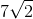and two equal angles.

Quantity B:Quantity B is greater.

The relationship cannot be determined from the relationship given.

Both quantities are equal.

Quantity A is greater.

Quantity B is greater.

Explanation:

The first step of a quantitative comparison question is to see if the quantities can even be determined in the first place.  The Quantity B is just a number, so it is already determined.  In Quantity A, you must find the perimeter of a right triangle with a given side-length and two equal angles.  This is determinable, since you have all three angles and a side length.

To solve Quantity A, first consider that two of the angles in the right triangle must be equal. As you cannot have multiple 90 degree angles in a triangle, then the two equal angles must be the other angles. This means they're both 45 degrees, and you have a 45-45-90 triangle.  This is a special triangle, where the ratio of side:side:hypotenuse is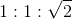.  Since the hypotenuse is equal to, then the side lengths are both.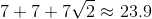Quantity B is greater.

Tired of practice problems?

Try live online GRE Math prep today.